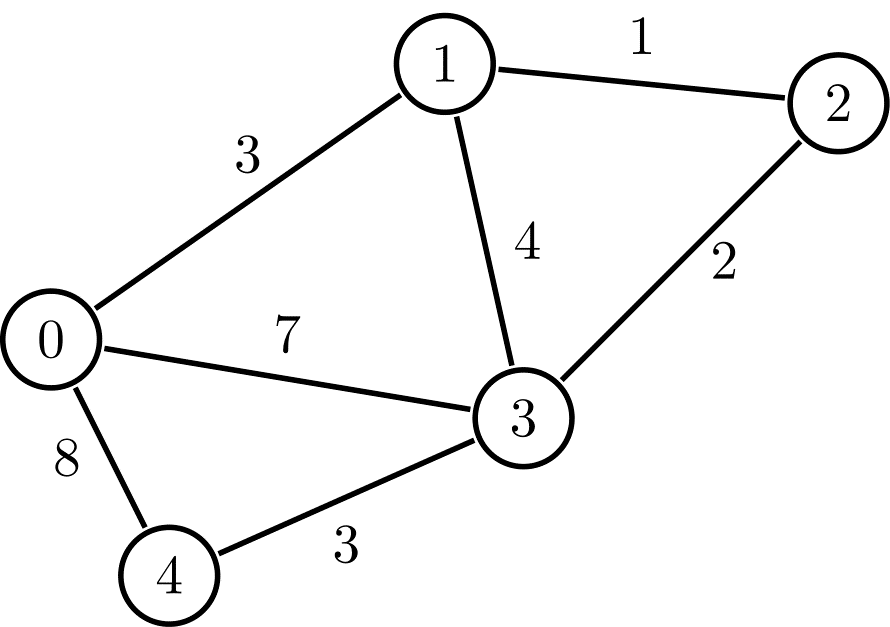Posted: 5 Dec, 2020
Difficulty: Easy

## PROBLEM STATEMENT

#### You live in the country with ‘V’ cities that have ‘E’ roads. You are in city ‘S’ with a car having ‘P’ amount of petrol in it. Roads are bidirectional and consume your petrol. Each road has a description ‘X’, ‘Y’ and ‘Z’, which means city ‘X’ and ‘Y’ have a road between them which consumes ‘Z’ amount of petrol. You want to visit the city ‘D’. Your task is to check if it's possible to visit ‘D’ from ‘S’ using ‘P’ amount of petrol.

##### For Example:
``````In the given graph, if we want to reach from node 1 to node 4 with ‘P’ = 10, then our answer is ‘Yes’ because there exists path 1 -> 3 -> 4, which takes 7 amount of petrol, which is less than the given value of ‘P’ so it is possible and hence, the answer is ‘Yes’.
``````##### Input Format:
``````The first line of the input contains a single integer, 'T,’ denoting the number of test cases.

The first line of each test case contains three space-separated integers, ‘V’, ‘E’, and ’P’, denoting the number of vertices, the number of edges in the graph, and the amount of petrol in the car.

The next ‘E’ lines of each test case contain three space-separated integers, ‘X’, ‘Y’ and ‘Z’, denoting the vertices which are connected with a bidirectional edge, and the weight of the edge.

The last line of each test case has two space-separated integers, ‘S’ and ’D’, representing the source and destination vertices.
``````
##### Output Format:
``````For each test case, print “Yes” if there exists a path from vertex 'S’ to 'D’ which consumes less than or equal to the given amount of petrol ‘P’. Otherwise, print “No” (without quotes).

Print the output of each test case in a separate line.
``````
##### Note:
``````You do not need to print anything, it has already been taken care of. Just implement the given function.
``````
##### Constraints :
``````1 <= T <= 10
2 <= V <= 2000
0 <= E <= (V*(V-1)) / 2
0 <= X,Y < V
1 <= Z <= 10 ^ 6
1 <= P <= 10 ^ 9
0 <= S,D < V

Time Limit: 1 sec
``````Approach 1

In this approach, we will calculate the shortest path between all pairs of vertices using the values of already computed values. This algorithm is known as Floyd Warshall Algorithm.

Floyd Warshall Algorithm is a dynamic programming approach in which we try to find the shortest distance of a pair of vertices using an intermediate vertex whose value is already calculated as -:

``````distance[index1][index2] = min(distance[index1][index2], distance[index1][k] + distance[k][index2])

Where k is the intermediate vertex.``````

Algorithm:

• Floyd Warshall Algorithm:
• Make an array distance of size (V)*(V) to store the shortest distance between all pairs of vertices.
• Initialize all values of the array by maximum integer value because, in the beginning, we assume that all vertices are unreachable.
• For all vertices index from 0 to V - 1.
• set distance[index][index] as 0 (distance between same vertices is 0).
• Set the values of the edge weights into distance from the given edges.
• Using each vertex from 0 to V - 1 as intermediate vertex k, do the following steps:
• For a pair between index1 and index2, we are trying to minimize its minimum distance using intermediate vertex k. We will iterate k from 0 to V - 1.
• Set distance[index1][index2]  as the minimum of distance[index1][index2] and distance[index1][k] + distance[k][index2].
• Values of the Shortest distance between each pair are calculated.
• Return True if the value of distance[S][D] (Shortest distance between S and D) is less than or equal to P. Otherwise, return False.